# What are the Different Types of Data?

Community of Thinkers & Writers
Oct 27, 2020 11:17 AM 7 min read
Editorial

A data driven viewpoint validates various decisions in the sphere of policy making and business. Before getting into the analysis, one must understand that data is gold and it requires to be graded precisely. Knowing the nature and type of data becomes a prerequisite to perform statistical analysis.

## What is a Survey?

Survey is a method of collecting data that can be done by anyone, the sample chosen will be surveyed to obtain the relevant information. Census, on the other hand is conducted only by government agencies and is the most reliable form of data collection because it collects general data from everyone. But it is almost impossible to collect detailed information via census because of the heterogeneity in the population. Population is an accurate example for census where almost every person in the country is reached out to collate generic data.

Let us assume we are interested in conducting primary research based on the research question framed. There are various modes of data collection that can be fit into our analysis depending on what the researcher wants to find out. To illustrate, our research question pertains to analyzing the graduate students’ learning feedback of the online lecture format. In this case, the target group would be all the students attending online lectures. But clearly, it would be impossible to collect data from every student, so we pick a sample of students and get the feedback through our questionnaire.

It is vital to know the types of data before framing the questionnaire and proceeding to the analysis. This serves to clarify the nature of data and type of analysis that could be employed. In our illustration, the sample population will be surveyed using a basic questionnaire about the questions relevant for the research study. Broadly, data can be qualitative and quantitative – it is usually advisable to use a mix of both data to arrive at more comprehensive results.

## Nominal Data

Certain label questions that have no numerical value are called as nominal data. In our example gender and the location of college are qualitative labels that have no number assigned to it. Such data is more representative but an exclusive statistical analysis on nominal data is not possible. Only frequency distribution and mode can be performed with a set of nominal data. For example, after the completion of the survey we will be able to find out the total frequency of  females and male who took the survey.

Mode is a measure of central tendency that captures the most frequently appearing item in the data set. Unlike Mean and Median, Mode has a single value assigned to a choice. Thus, it simply looks at the most commonly occurring frequency in our dataset. In simple words, nominal data are abstract questions with no intrinsic numerical value. Nominal scale can be graphically represented in the form of pie chart, bar graph, etc.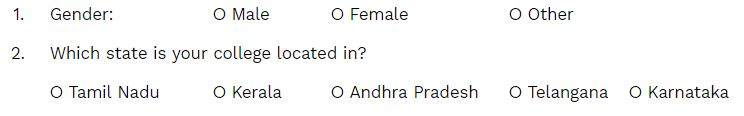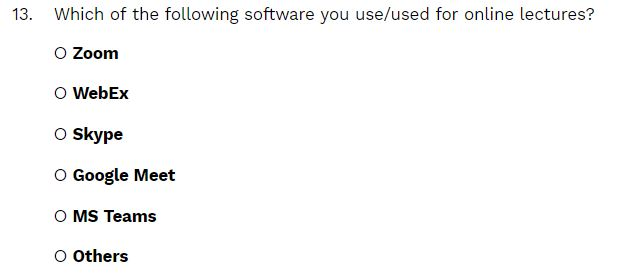Above is another example of a nominal data question that tried to get a simple label information about which application students used to attend lectures.

## Ordinal Data

Like the name suggests, ordinal is to order the discrete choices. It is very similar to nominal except the order of the options actually matters. So think of all the customer satisfaction surveys you have taken before, it falls under the ordinal category. The order of the option is relevant to perform any type of statistical analysis. In our research example, since it is a learners’ feedback survey trying to get the perception of students, most of the questions are ordinal in nature. The psychometric questions are designed to collect the ordinal data.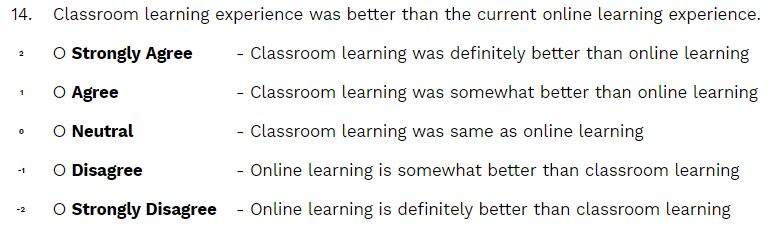Although the choices are in text, while decoding the data, a numerical value is given to each option in an order. For example, if neutral has the value 0 (because the student might feel online learning experience is the same as classroom learning experience) the other options would carry values as listed below: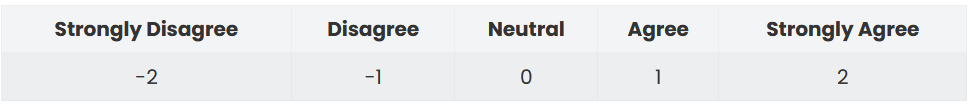After receiving the response from students, we can construct a frequency distribution table to find out the frequency of students who are neutral with the statement. If the maximum number of students choose neutral – this would mean a large chunk of students feel that their online experience is the same as classroom

But the biggest limitation of using ordinal scale is that it can only order the preference, the question simply obtains the perception. The perceived difference between strongly agree and agree might not be the same as disagree to strongly disagree. In other words, the student’s idea of strongly agree might be more prominent with respect to agree, however, same prominence cannot be expected between strongly disagree and disagree.  Due to this character of data, most of the complex statistical analysis cannot be performed with ordinal data. Only qualitative aspects of the sample are constructed through an ordinal data format.

Ordinal scale cannot be represented in a Pie chart, it can be visualized in column chart or bar graph.

## Interval

Interval data relates to quantitative data that has a defined numerical value. The data can be either discrete or continuous, discrete data means they can be measured as they are counted. Continuous data can be represented with an upper limit and lower limit number that cannot be counted as measured.

One question under learning environment assessment pertains to the number of students in the family. This is an example of interval scale data – under this type “zero” holds no value. That means, true zero would not be possible since there is definitely at least one student in the family and it is technically impossible to have zero students since we are surveying students.Other popular examples of interval scale are, temperature and time. Mean, Median, Mode and frequency distribution can be done with the interval data.

## Ratio

Ratio scale is the type of data which can adapt with any statistical and inferential analysis. It is basically an ordered data and interval scale with a true value of zero.

The difference and proportion between two ratios is the same and consistent. In our example, we have a question asking the number of hours a student spends doing domestic tasks. The data collected would be a ratio scale, the difference between each choice is one hour which is consistent in all the options. This type of scale is the most versatile one and the data can be represented in box plot or histogram.

Why is Ratio scale better?

The most significant difference between ratio scale and others is that all measures of central tendency and geometric mean can be performed in this case. In geometric mean, we take the product of our data and square it instead of taking the sum as we do in Arithmetic mean (AM) . Basically, we can conclude an average number of hours spent by a student using AM and a multiplicative scaling factor – something more advanced in giving us a better picture since it accounts for skewed dataset (A separate write up on GM is warranted).

The continuous data offers the researcher to represent the responses better, for example an average number of hours being spent on domestic work can be calculated. Moreover, this measurement is not just quantitative but also has a true value of zero. Four hours of domestic work is clearly twice as more than two hours so multiplication and division is also possible with this scale. Due to the consistency offered within the scale, coefficient of variation is also available. It measures the variability in a data and stabilizes the volatility that arises because the data is  skewed. By using coefficient of variation, ratio type data takes the standard deviation and divides the same with the mean. This is not possible in interval scale because it is a discrete data set with no true zero. Multiplication and division would not make sense in the case of interval scale and so does coefficient of variation.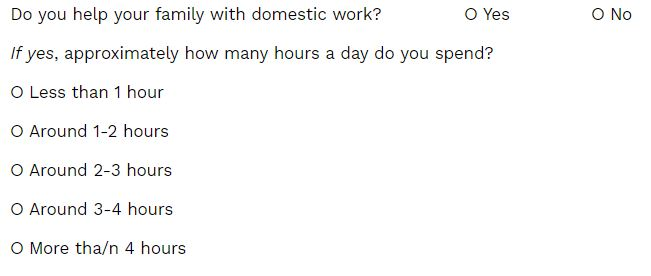We can conclude that Ratio is the best of all, but a questionnaire has to be framed based on the research question. Understanding the types of scale and data will enable the researcher to optimize the questionnaire in the most suitable format to produce meaningful statistical analysis. A combination of both qualitative and quantitative is preferred in primary surveys. Appropriate data visualization has to be undertaken to accurately present all the data collected at the end of the study.